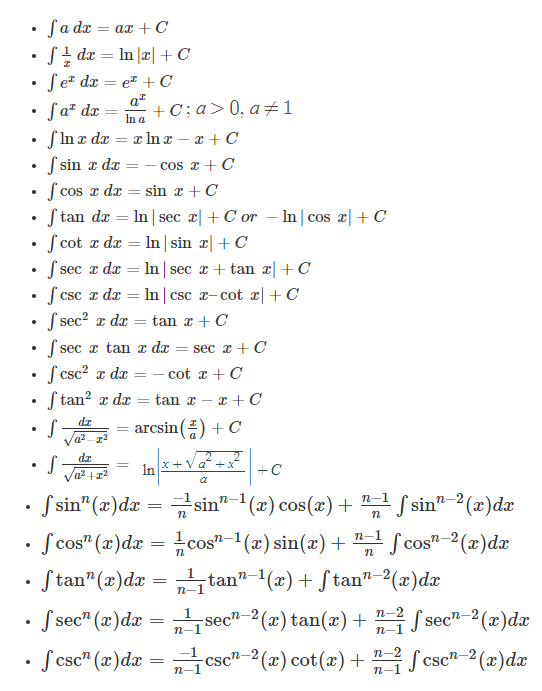# Integral

In mathematics, an integral plays a crucial role while dealing with calculus concepts. It assigns numbers to functions to express displacement, area, volume and other theories that arise by connecting infinitesimal data. However, there exist several applications of integrals in various fields. In this article, you will learn what integral means. Types of integrals, formulas and integrals for some of the functions in detail.

## Integral Meaning

The most common meaning of an integral is calculus’s real object corresponding to summing tiny pieces to find a continuous region’s content. Integration is the process of computing an integral and a more obsolete term for integration is quadrature. The approximate estimate of an integral is known as numerical integration. Also, integrals can be classified into two types, namely definite and indefinite integrals.

### Definite Integral

An integral that contains the upper and lower limits (i.e., start and end value) is known as a definite integral. The numerical of x is restricted to lie on the real line. A definite integral is written as:

$$\int_{a}^{b} f(x) dx$$

Here,

f(x) = A function of real variable x,

a, b = limits (the interval [a, b] on the real line]

This can be simplified as:

$$\int_{a}^{b} f(x) dx = [F(x)]_{a}^{b}=F(b) – F(a)$$

Definite Integral is also called a Riemann Integral when it is determined to lie on the real line.

### Indefinite Integral

The indefinite integrals represent the family of the given function whose derivatives are functions. It yields a function of the independent variable after integration. Indefinite integrals are not determined using the upper and lower limits.

The integration of a function f(x) is given by F(x) and it is written as

∫f(x) dx = F(x) + C

Where,

F(x) + C of the equation means integral of f(x) with respect to x

F(x) is called an anti-derivative or primitive function.

f(x) is called the integrand.

dx is called the integrating agent.

C is called the constant of integration.

x is the variable of integration.

The operation of integration, up to an additive constant, is the inverse of differentiation operation. For this reason, the term integral may also refer to the related notion of the antiderivative. Thus, a function F or F(x) whose derivative is the given function f or f(x).

## Integral Formulas

Some of the important formulas of integral functions are listed below:### Integral uv Formula

The uv formula of integral is generally used to calculate the integration by parts. This can be expressed as:

$$\int du(\frac{dv}{dx})dx=uv-\int v(\frac{du}{dx})dx$$

Here,

u = Function of u(x)

v = Function of v(x)

dv = Derivative of v(x)

du = Derivative of u(x)

### Integral log x

Let us derive the formula for integral log x here.

∫log x dx

We know that,

∫ uv′ = uv – ∫ vu′ where u and v are functions of x and prime here indicates derivatives.

Using the ILATE rule, let us take the functions as u(x) and v(x).

u(x)= log x and v(x)= x

Thus, by substituting these functions in the formula we get,

∫log x dx = x(log x) – ∫x.(dx/x) + C

= x(log x) – ∫dx + C

= x(log x) – x + C

Where C is integration constant.

### Integral of tan x

The integral of tan x can be derived using substitution method. Here, we have to assume one part of the function as u and find the derivative of this function to substitute in the integral of the given function. This can be understood in a better way using the derivation given below.

∫tan x dx

Let cos x = u

-sinx dx = du

sin x dx = -du

∫tan x dx

= ∫(sin x/cos x) dx

= -∫du/u

= -ln u + C

=-ln cos x + C

Or

= ln sec x + C

Therefore, ∫tan x dx = -ln (cos x) + C = ln (sec x) + C